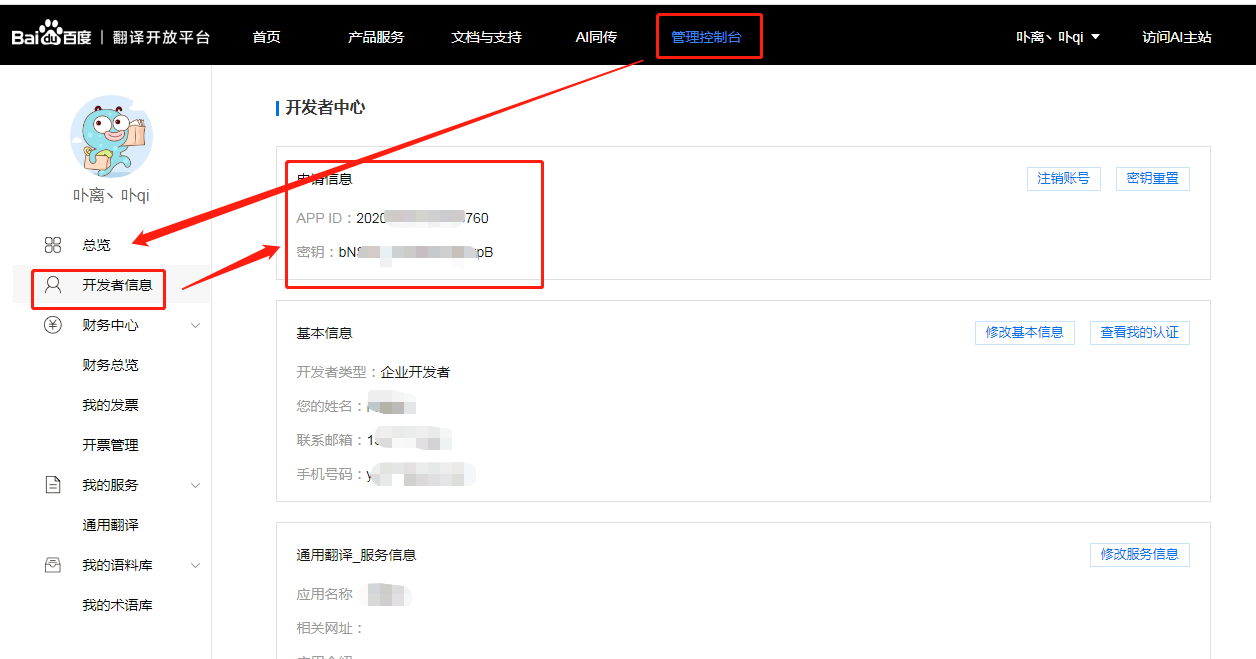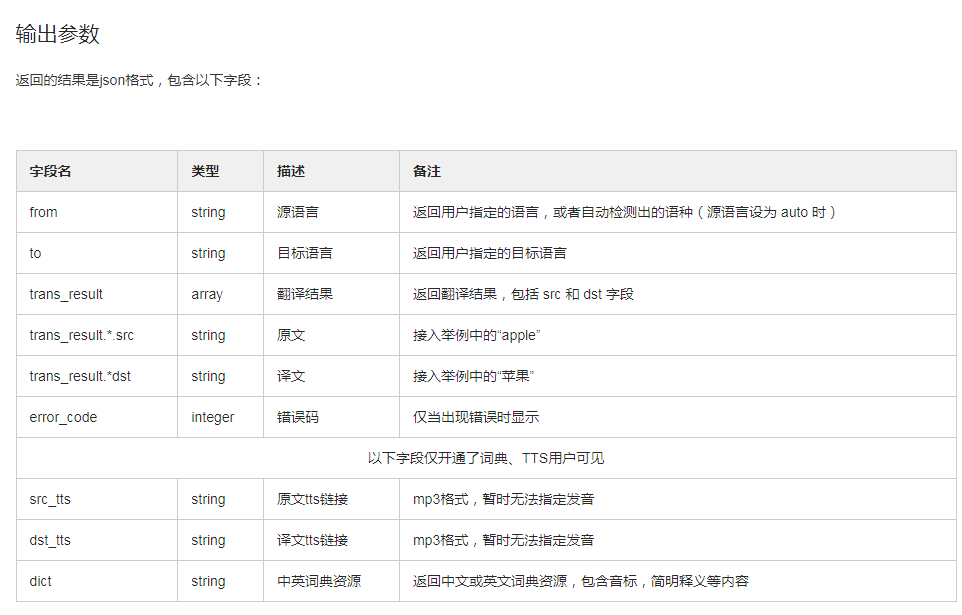PHP使用百度翻译API接口将中文翻译成英文

2021-08-03 15:49 174 梁俊威

PHP开发时，大多数外贸网站会出现需要使用翻译接口的时候，今天这篇文章主要讲解如何使用百度翻译API接口翻译中文。public function index(){
if(empty(\$this->input['key'])){
exit(json_encode(["code"=>0,'msg'=>"请输入单词"]));
}
\$key = \$this->input['key'];
if(!preg_match('/^[\x{4e00}-\x{9fa5}]+\$/u', \$key)>0){
exit(json_encode(["code"=>0,'msg'=>"请输入中文，且不能有特殊符号"]));
}
\$array = \$this->language(\$key);

//开始执行你的逻辑代码

}

// 封装好的调用方法
protected function language(\$q,\$from="zh",\$to="en"){
//您注册的API Key
\$appid="";
\$secret = "";
// 随机撒盐值
\$salt = rand(100000, 999999);

// 签名
\$sign = md5(\$appid.\$q.\$salt.\$secret);

//首先对要翻译的文字进行 urlencode 处理
\$q=urlencode(\$q);

// 百度翻译API
\$url = "https://fanyi-api.baidu.com/api/trans/vip/translate";

\$url .= "?q=".\$q;
\$url .= "&&from=".\$from;
\$url .= "&&to=".\$to;
\$url .= "&&appid=".\$appid;
\$url .= "&&salt=".\$salt;
\$url .= "&&sign=".\$sign;
\$url .= "&&tts=0";

\$file_contents = file_get_contents(\$url);
return json_decode(\$file_contents, true);
}相关推荐

• PHP调用百度人脸识别姓名身份证检测